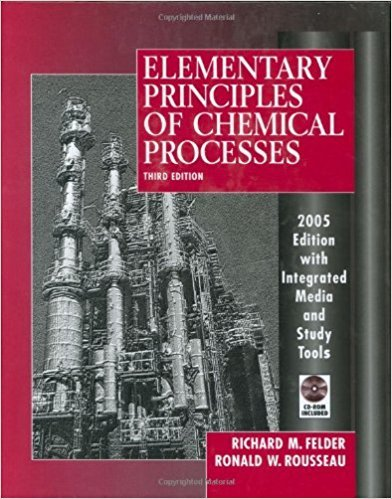×
Get Full Access to Elementary Principles Of Chemical Processes - 3 Edition - Chapter 8 - Problem 8.45
Get Full Access to Elementary Principles Of Chemical Processes - 3 Edition - Chapter 8 - Problem 8.45

×

# A stream of pure cyclopentane vapor flowing at a rate ofISBN: 9780471687573 143

## Solution for problem 8.45 Chapter 8

Elementary Principles of Chemical Processes | 3rd Edition

• Textbook Solutions
• 2901 Step-by-step solutions solved by professors and subject experts
• Get 24/7 help from StudySoup virtual teaching assistantsElementary Principles of Chemical Processes | 3rd Edition

4 5 1 275 Reviews
18
0
Problem 8.45

A stream of pure cyclopentane vapor flowing at a rate of 1550 L Is at 150D C and 1 atm enters a cooler in which 55% of the feed is condensed at constant pressure. (a) What is the temperature at the condenser outlet? Explain how you know (a single sentence should suffice). (b) Prepare and fill in an inlet-outlet enthalpy table and calculate the required cooling rate in kW

Step-by-Step Solution:
Step 1 of 3

Chapter 6: Energy Relationships in Chemical Reactions Energy is described as the capacity to do work Types of Energy 1. Radiant energy comes from the earth’s primary energy source, the sun 2. Thermal energy is the energy associated with the random motion of atoms and molecules 3. Chemical energy is the energy stored in the bonds of chemical substances 4. Nuclear energy is the energy stored within the neutrons and protons in the atom 5. Potential energy is the energy available by virtue of an object’s position Heat is the transfer of thermal energy between two bodies at different temperatures Thermochemistry: The study of heat change in chemical reactions The sys

Step 2 of 3

Step 3 of 3

##### ISBN: 9780471687573

This full solution covers the following key subjects: outlet, rate, inlet, condenser, constant. This expansive textbook survival guide covers 13 chapters, and 710 solutions. Since the solution to 8.45 from 8 chapter was answered, more than 244 students have viewed the full step-by-step answer. The full step-by-step solution to problem: 8.45 from chapter: 8 was answered by , our top Chemistry solution expert on 11/15/17, 02:42PM. Elementary Principles of Chemical Processes was written by and is associated to the ISBN: 9780471687573. The answer to “A stream of pure cyclopentane vapor flowing at a rate of 1550 L Is at 150D C and 1 atm enters a cooler in which 55% of the feed is condensed at constant pressure. (a) What is the temperature at the condenser outlet? Explain how you know (a single sentence should suffice). (b) Prepare and fill in an inlet-outlet enthalpy table and calculate the required cooling rate in kW” is broken down into a number of easy to follow steps, and 69 words. This textbook survival guide was created for the textbook: Elementary Principles of Chemical Processes, edition: 3.

Unlock Textbook Solution